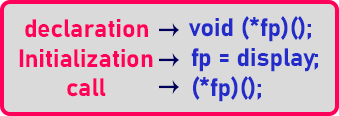# Function Pointer in C++

## Function Pointer in C++ with Examples:

In this article, I am going to discuss Function Pointer in C++ Language with examples. Please read our previous articles, where we discussed References in C++ with examples.

##### Function Pointer in C++

We know well that we can have a pointer to any data type. For any primitive data type, we can have a pointer of the type class. Now can we have a pointer to a function? Yes.

```void display(){
cout << "Hello"'
}
int main(){
void (*fp)();
fp = display;
(*fp)();
}```

We have one function here ‘display ()’ and some statements in the main function. The function ‘display’ is not taking any parameter and does not return anything. Now we want to call the ‘display’ function from the main function. If we have to call this function we can simply write ‘display ()’ in the main function and ‘Hello’ will be displayed.

This function is called and ‘hello’ is displayed and the control returns back. We know this, now instead of directly calling the name, can we use a pointer to a function? Yes. So let us see that.

We will define a pointer to a function and will call that pointer ‘fp’. We will write it as ‘void (*fp)();’. The return type is ‘void’ and there are no parameters so leave parenthesis empty. So, this is the method of declaring a pointer to a function and when you declared a pointer to a function it must be inside the brackets i.e. (*fp) otherwise it will not be a pointer to a function.

After the declaration of a pointer, we will be assigned ‘fp’ to ‘display’ as ‘fp = display’. Now this will be assigned the name of a function. So, the address of that function will be stored in this pointer. This is the initialization of the pointer to a function. Now how to call this? We can call it as, ‘(*fp)()’.This is how a pointer can be assigned to a function. Now let’s see one more example.

```int max(int x, int y){
return x > y ? x : y;
}
int min(int x, int y){
return x < y ? x : y;
}
```

So, we have two functions here ‘max’ and ‘min’. Both are taking two integers as parameters and ‘max’ is for finding the maximum of two integers and ‘min’ is for finding the minimum of two integers.

```int main(){
int (*fp)(int, int);
fp = max;
(*fp)(10, 5);
fp = min;
(*fp)(10, 5);
}
```

Now inside the main function, we have declared a pointer to function as ‘int (*fp)(int, int)’ as our ‘max’ and ‘min’ function takes two integers as parameters so we have to define that prototype at time of declaration of a pointer to function.

Next, we assign ‘fp’ to ‘max’. Here ‘fp’ is holding the address of the ‘max’ function. And on the next line, we call the ‘max’ function as ‘(*fp)(10, 5)’. Here we gave ‘10’ and ‘5’ as parameters.

Next, we modified ‘fp’ as assigned to ‘min’. Here ‘fp’ is holding the address of the ‘min’ function. Then we call the ‘min’ function as ‘(*fp)(10, 5)’.

So, we call a different function by pointing our pointer to different functions. This is the same name for different functions or different operations. This is just like polymorphism. In function overriding, internally function pointer is used for achieving a runtime polymorphism using function overriding. So, this means that one function pointer can point to any function which is having the same signature.